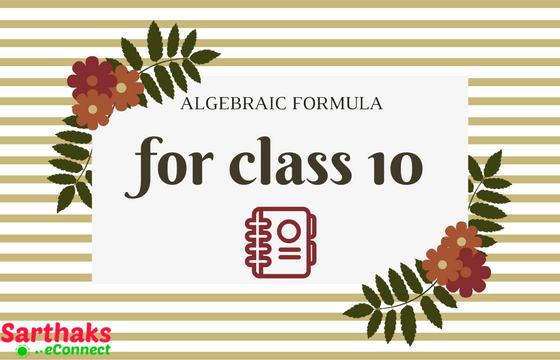### Algebra Formula for class 10:

Algebra is a major part of mathematics which has use of letters and symbols for representation of numbers. In this section we have provided you with algebra formula for class 10. It acts as a solving entity for algebraic equations all over. This portion has a great significance in mathematics from basics up to the research level. Algebra is categorized into two parts as Elementry algebra and Modern algebra (also known as Abstract algebra). We at this stage deal with only elementary algebra which contains basics of algebra and use of its formula. For the professionals who research in algebra section of mathematics studies abstract algebra.

Elementary algebra generally contains calculative part and solution based equations.

In class 10th, linear equation with single variable and with two variables, quadratic equation chapters, you will need algebraic procedures to solve equations. There in instance, you will see several problems with variables go accordingly and need high expressions of equations.

It is much necessary for you to learn all the formula before studying this chapter and also remember what you have studied in class 9th. Recall it, however, we have taken all the formulas of algebra and related topics so that it will be easy for you to learn and recall. Otherwise, you should search the chapters and collect them from everywhere but happily, we have done this work for you.

## There is list of all algebra formula you will need in solving problems in this class. Learn it.

 (a+b)2 = a2+2ab+b2 (a−b)2 = a2−2ab+b2 (a+b)(a–b) = a2–b2 (x+a)(x+b) = x2+(a+b)x+ab (x+a)(x–b) = x2+(a–b)x–ab (x–a)(x+b) = x2+(b–a)x–ab (x–a)(x–b) = x2–(a+b)x+ab (a+b)3 = a3+b3+3ab(a+b) (a–b)3 = a3–b3–3ab(a–b) (x+y+z)2 = x2+y2+z2+2xy+2yz+2xz (x+y–z)2 = x2+y2+z2+2xy–2yz–2xz (x–y+z)2 = x2+y2+z2–2xy–2yz+2xz (x–y–z)2 = x2+y2+z2–2xy+2yz–2xz x3+y3+z3–3xyz = (x+y+z)(x2+y2+z2–xy–yz−xz) x2+y2 = 1/2[(x+y)2+(x–y)2] (x+a)(x+b)(x+c) = x3+(a+b+c)x2+(ab+bc+ca)x+abc x3+y3 = (x+y)(x2–xy+y2) x3–y3 = (x–y)(x2+xy+y2) x2+y2+z2−xy–yz–zx = 1/2[(x−y)2+(y−z)2+(z−x)2]

Learn the algebra formula for class 10 to score better in your examinations. This formula sheet is not only beneficial for CBSE board class 10 but also for SSC, Railway and Banking examinations.